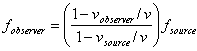Physics 101 Formulas

Kinematics
vave = Dx/Dt          aave = Dv/Dt
v = v0 + at                 x = x0 + v0t + 1/2at2         v2 = v02 + 2aDx
g=9.81m/s2 = 32.2ft/s2 (near Earth’s surface)

Dynamics
SF = ma                 Fg = Gm1m2 / R2            Fg = mg (near Earth’s surface)
fs,max = msFN           Gravitational constant, G = 6.7 x 10-11 N.m2/kg2
fk = mkFN                       ac = v2 / R = w2R

Work & Energy
WF = FScos(q)        KE = 1/2mv2      WNET = DKE = KEf - KEi
Wnc = DE = Ef - Ei = (KEf + PEf) - (KEi + PEi)
Wgrav = -mgDy   PEgrav = mgy

Impulse & Momentum
Impulse I = FaveDt = Dp      FaveDt = Dp = mvf - mvi           Fave = Dp/Dt
SFextDt= DPtotal  = Ptotal,final- Ptotal,initial     (momentum conserved if SFext = 0)
Xcm = (m1x1+ m2x2)/ (m1+m2)

Rotational Kinematics
w = w0 + at               q = q0 + w0t + 1/2at2       w2 = w02 + 2aDq
vT = wR    aT = aR   ( so for rolling without slipping v = wR    a = aR )

Rotational Statics & Dynamics
t = Fr sin q
St = 0 and SF=0  (static equilibrium)
St = Ia
I = Smr2   (for a collection of point particles)
I = 1/2MR2 (solid disk or cylinder)   I = 2/5MR2 (solid sphere)   I = 2/3MR2 (hollow sphere)
I = MR2 (hoop or hollow cylinder)     I = 1/12 ML2 (uniform rod about center)
W = tq    (work done by a torque)
L =Iw        StextDt = DL     (angular momentum conserved if Stext = 0)
KErot=1/2Iw2 =L2/2I     KEtotal=KEtrans + KErot = 1/2mv2 + 1/2Iw 2

Simple Harmonic Motion
Hookes Law:  Fs = -kx
Wspring = 1/2kxi2 - 1/2kxf2   PEspring = 1/2kx2
x(t) = Acos(wt)   or   x(t) = Asin(wt)
v(t) = -Awsin(wt)   or   v(t) = Awcos(wt)
a(t) = -Aw2cos(
wt)   or   a(t) = -Aw2sin(wt)
w2 = k/m,            T = 2p/w,        f = 1/T
xmax= A        vmax = wA      amax= w2A
For a simple pendulum  w 2 = g/L

Fluids
P2 = P1 + rg(y1-y2)  change in pressure with depth
Buoyant force FB = rgVdis = weight of displaced fluid
Flow rate Q = v1A1 =  v2A2      continuity equation   (area of circle A = pr2)
P1 + 1/2rv12 + rgy1 = P2 + 1/2rv22 + rgy2    Bernoulli equation
rwater = 1000 kg/m3                   1m3 = 1000 liters
r = M/V                            1 atmos. = 1.01 x 105 Pa

Temperature and Heat
temperature:
Celsius (TC) to Fahrenheit (TF) conversion: TC=(5/9)*(TF-32)
Celsius (TC) to Kelvin (TK) conversion: TK=TC+273
DL = aL0DT       DV = bV0DT      thermal expansion
aaluminum = 23 x10-6 and asteel = 12 x10-6 (linear expansion coefficients)
Q = cMDT  specific heat capacity
cwater = 4186 J/kg/oC   cice = 2000 J/kg/oC
caluminum = 900 J/kg/oC   csteel = 450 J/kg/oC
Q = LfM   latent heat of fusion           Q = LvM   latent heat of vaporization
Lf,water = 33.5 x104 J/kg     Lv,water = 22.6 x105 J/kg
ksteel = 14 J/s/m/oC     kaluminum = 240 J/s/m/oC  (thermal conductivities)
Q = esT4At      radiation      (s = 5.67x10-8 J/s/m2/oK4)
Pnet = esA(T4-T04)            (surface area of a sphere A = 4pr2 )

Ideal Gas & Kinetic Theory
NA=6.022 x 1023   molecules/mole        Mass of carbon-12 = 12.000u
PV = nRT = NkBT      R = 8.31 J/mol/K      kB = R/NA = 1.38 x 10-23 J/K
KEave = 3/2kBT =  1/2mvrms2       U =  3/2NkBT (internal energy of a monatomic ideal gas)
vrms2   =  3kBT/m   =  3RT/M  (M = molar mass = kg/mole)

Thermodynamics
Q = DU + W  (1'st law)

U =  (3/2)nRT   (internal energy of a monatomic gas for fixed n)

CV  = (3/2)nR = 12.5 J/oC/mol   (specific heat at constant volume for a monatomic gas)

QH = QC + W  (heat engine or refrigerator)
e = W/QH = 1 - QC/QH     emax = 1 - TC/TH (Carnot engine)
QC/QH = TC/TH   when efficiency is maximum (2'nd law)
W = PDV  (work done by expanding gas)

Harmonic Waves
v  =  l / T = l f
v2 = F/(m/L)  for wave on a string
v2 = 1.4kBT/m for sound
v = c = 3 x 108 m/s  for electromagnetic waves (light, microwaves, etc.)
I = P / (4pr2)  (sound intensity)

Sound Waves
loudness: β=(10 dB) log10 (I/I0), where I0=10-12 W/m2(
Doppler effect)